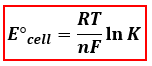# Problem: Calculate the standard potential, E°, for this reaction from its equilibrium constant at 298 K.X(s) + Y2+(aq) ⇌ X2+(aq) + Y(s)   K= 9.49 x 105

###### FREE Expert Solution
83% (415 ratings)
###### FREE Expert Solution

We are asked to calculate for the standard potential (E°cell) of the reaction. We will use the Nernst Equation to calculate for the ratio. The Nernst Equation relates the concentrations of compounds and cell potential.E°cell = cell potential, V
R = gas constant = 8.314 J/(mol
·K)
T = temperature, K
n = mole e- transferred
F = Faraday’s constant, 96485 C/mol e-
K = equilibrium constant

Let’s first determine how many electrons were transferred in the reaction:

83% (415 ratings)###### Problem Details

Calculate the standard potential, E°, for this reaction from its equilibrium constant at 298 K.

X(s) + Y2+(aq) ⇌ X2+(aq) + Y(s)   K= 9.49 x 105